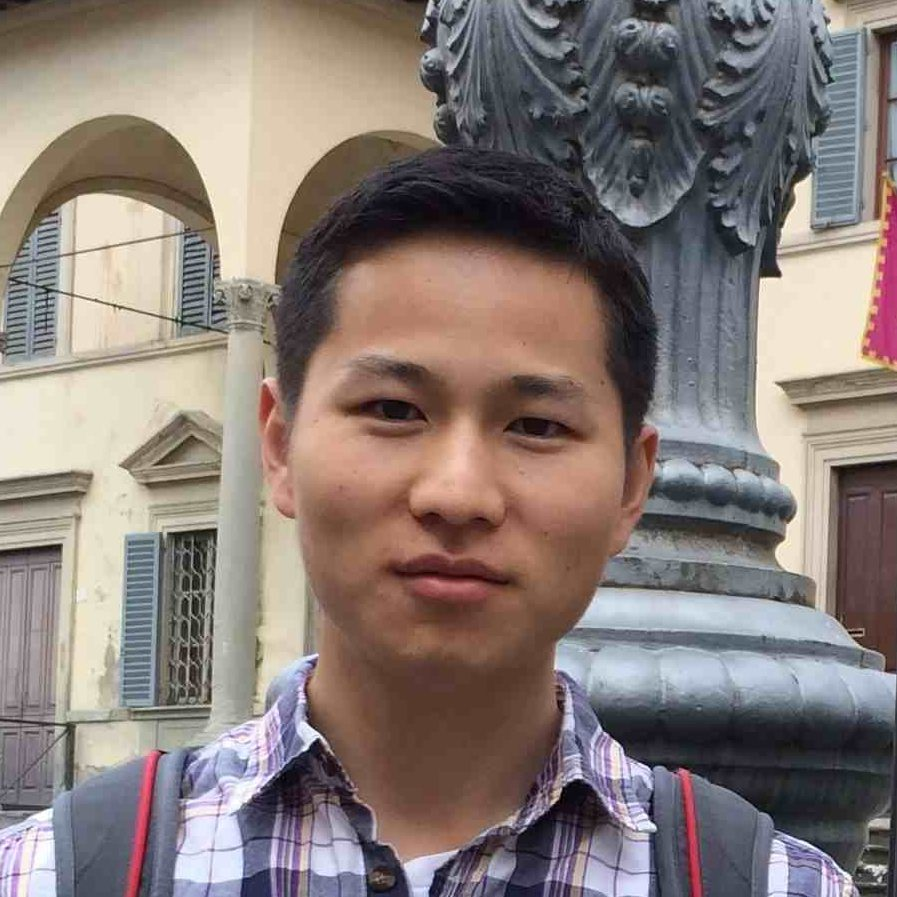Zhizhang Xie
(Texas A&M University)
February 11, 2022
15:00
(EST)
Organized by Americas GNCG Seminar.

## On Gromov’s Dihedral extremality and rigidity conjectures

In this talk, I will present my recent joint work with Jinmin Wang and Guoliang Yu on Gromov’s dihedral extremality and rigidity conjectures. These two conjectures of Gromov concern comparisons of scalar curvature, mean curvature and dihedral angles for compact manifolds with corners, which can be viewed as scalar curvature analogues of the Alexandrov’s triangle comparisons for spaces whose sectional curvature is bounded below. Gromov’s conjectures have profound implications in geometry and mathematical physics such as the positive mass theorem. In our recent work, by using Dirac operator methods, we answer positively Gromov’s dihedral extremality conjecture for convex polyhedra in all dimensions, and Gromov’s dihedral rigidity conjecture for convex polyhedra in dimension three.